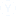what is shortcut for solving ratio and proportion
Solving ratio and proportion problems using shortcuts
• GS
• Gokul
• 01 अक्तूबर
• 721 दृश्य
• 1 उत्तर1 उत्तर
1-1 of  1
• u want a trick for solving Ratio and Proportion method... Its so easy Friend
u have to remmber some points always for exmple Types of Ratio:-
Duplicate ratio: The ratio of the squares of the two numbers.
Ex: 9 : 16 is the duplicate ratio of 3 : 4.

2. Triplicate Ratio: The ratio of the cubes of the two numbers.
Ex: 27 : 64 is the triplicate ratio of 3 : 4.
3. Sub-duplicate Ratio: The ratio between the square roots of the two numbers.
Ex: 4 : 5 is the sub-duplicate ratio of 16 : 25.

4. Sub-triplicate Ratio: The ratio between the cube roots of the two numbers.
Ex: 4 : 5 is the sub-triplicate ratio of 64 : 125.

5.Inverse ratio: If the two terms in the ratio interchange their places, then the new ratio is inverse ratio of the first.
Ex: 9 :5 is the inverse ratio of 5 : 9.

6. Compound ratio: The ratio of the product of the first terms to that of the second terms of two or more ratios.
Ex: The compound ratio of  3/4, 5/7, 4/5, 4/5 is 9/35

Now As like Ratio There will be Type of Proportion also let us see them also :-
Continued Proportion: In the proportion  8/12= 12/8  8, 12, 18 are in the continued proportion.

Fourth proportion: If a : b = c : x, then x is called fourth proportion of a,b and  c.
There fore fourth proportion of  a, b, c  = b x c/a

Third proportion: If a : b = b : x, then x is called third proportion of a and b.
Therefore third proportion of a, b =  b^2/a

Second or mean proportion: If a : x = x : b , then x is called second or mean proportion of a and b.
Therefore mean proportion of a and b =  root(ab)अन्य संबंधित चर्चाएंमॉक परीक्षण अभ्यास के लिए
IBPS PO Prelim Exam